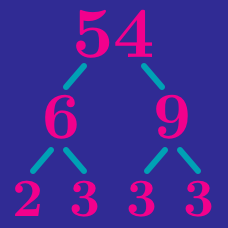Number Theory

# Number of Factors / Divisors

How many positive divisors does the number $2^63^8$ have?

How many factors does $2500000$ have?

Find the sum of all the positive divisors of 28.

How many positive integers less than or equal to $500$ have exactly $3$ positive divisors?

How many factors does the integer $4^8\times6^7\times12^7$ have?

×